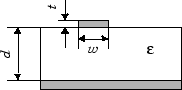Documentation

# Microstrip Transmission Line

Model microstrip transmission line

## Library

Transmission Lines sublibrary of the Physical library## Description

The Microstrip Transmission Line block models the microstrip transmission line described in the block dialog in terms of its frequency-dependent S-parameters. A microstrip transmission line is shown in cross-section in the following figure. Its physical characteristics include the microstrip width (w), the microstrip thickness (t), the substrate height (d), and the relative permittivity constant (ε).The block lets you model the transmission line as a stub or as a stubless line.

### Stubless Transmission Line

If you model a microstrip transmission line as a stubless line, the Microstrip Transmission Line block first calculates the ABCD-parameters at each frequency contained in the modeling frequencies vector. It then uses the `abcd2s` function to convert the ABCD-parameters to S-parameters.

The block calculates the ABCD-parameters using the physical length of the transmission line, d, and the complex propagation constant, k, using the following equations:

`$\begin{array}{l}A=\frac{{e}^{kd}+{e}^{-kd}}{2}\\ B=\frac{{Z}_{0}*\left({e}^{kd}-{e}^{-kd}\right)}{2}\\ C=\frac{{e}^{kd}-{e}^{-kd}}{2*{Z}_{0}}\\ D=\frac{{e}^{kd}+{e}^{-kd}}{2}\end{array}$`

Z0 and k are vectors whose elements correspond to the elements of f, a vector of modeling frequencies. Both can be expressed in terms of the specified conductor strip width, substrate height, conductor strip thickness, relative permittivity constant, conductivity, and dielectric loss tangent of the microstrip line, as described in .

### Shunt and Series Stubs

If you model the transmission line as a shunt or series stub, the Microstrip Transmission Line block first calculates the ABCD-parameters at each frequency contained in the vector of modeling frequencies. It then uses the `abcd2s` function to convert the ABCD-parameters to S-parameters.

### Shunt ABCD-Parameters

When you set the Stub mode parameter in the mask dialog box to `Shunt`, the two-port network consists of a stub transmission line that you can terminate with either a short circuit or an open circuit as shown here.Zin is the input impedance of the shunt circuit. The ABCD-parameters for the shunt stub are calculated as

`$\begin{array}{c}A=1\\ B=0\\ C=1/{Z}_{in}\\ D=1\end{array}$`

### Series ABCD-Parameters

When you set the Stub mode parameter in the mask dialog box to `Series`, the two-port network consists of a series transmission line that you can terminate with either a short circuit or an open circuit as shown here.Zin is the input impedance of the series circuit. The ABCD-parameters for the series stub are calculated as

`$\begin{array}{c}A=1\\ B={Z}_{in}\\ C=0\\ D=1\end{array}$`

## Parameters

### Main Tab

Strip width (m)

Width of the microstrip transmission line.

Substrate height (m)

Thickness of the dielectric on which the microstrip resides.

Strip thickness (m)

Physical thickness of the microstrip.

Relative permittivity constant

Relative permittivity of the dielectric expressed as the ratio of the permittivity of the dielectric to permittivity in free space ε0.

Loss tangent in dielectric

Loss angle tangent of the dielectric.

Conductivity in conductor (S/m)

Conductivity of the conductor in siemens per meter.

Transmission line length (m)

Physical length of the transmission line.

Stub mode

Type of stub. Choices are `Not a stub`, `Shunt`, or `Series`.

Termination of stub

Stub termination for stub modes `Shunt` and `Series`. Choices are `Open` or `Short`. This parameter becomes visible only when Stub mode is set to `Shunt` or `Series`.

### Visualization Tab

For information about plotting, see Create Plots.

## References

 Gupta, K.C., G. Ramesh, I. Bahl, and P. Bhartia, Microstrip Lines and Slotlines, Second Edition, Artech House, 1996. pp. 102-109.